## Example Questions

← Previous 1

### Example Question #56 : Coordinate Geometry

What is the equation of the straight line passing through (–2, 5) with an x-intercept of 3?

y = –x – 3

y = –x + 3

y = –5x – 3

y = –5x + 3

y = –x + 3

Explanation:

First you must figure out what point has an x-intercept of 3. This means the line crosses the x-axis at 3 and has no rise or fall on the y-axis which is equivalent to (3, 0). Now you use the formula (y y1)/(x– x1) to determine the slope of the line which is (5 – 0)/(–2 – 3) or –1. Now substitute a point known on the line (such as (–2, 5) or (3, 0)) to determine the y-intercept of the equation y = –x + b. b = 3 so the entire equation is y = –x + 3.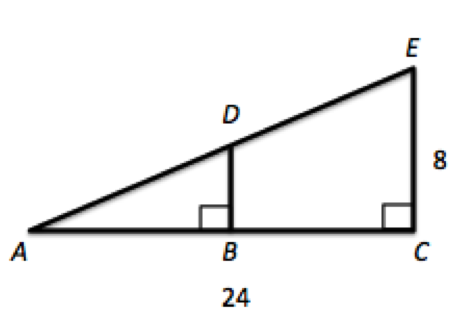### Example Question #1 : How To Find The Equation Of A Line

What is the equation of the line passing through (–1,5) and the upper-right corner of a square with a center at the origin and a perimeter of 22?

y = –x + 5

y = (3/5)x + 22/5

y = (–3/5)x + 22/5

y = (–3/5)x + 28/5

y = (–1/5)x + 2.75

y = (–3/5)x + 22/5

Explanation:

If the square has a perimeter of 22, each side is 22/4 or 5.5.  This means that the upper-right corner is (2.75, 2.75)—remember that each side will be "split in half" by the x and y axes.

Using the two points we have, we can ascertain our line's equation by using the point-slope formula. Let us first get our slope:

m = rise/run = (2.75 – 5)/(2.75 + 1) = –2.25/3.75 = –(9/4)/(15/4) = –9/15 = –3/5.

The point-slope form is: y – y0 = m(x – x0). Based on our data this is: y – 5 = (–3/5)(x + 1); Simplifying, we get: y = (–3/5)x – (3/5) + 5; y = (–3/5)x + 22/5

### Example Question #1 : How To Find The Equation Of A Line

What is the equation of a line passing through the points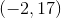and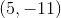?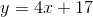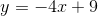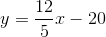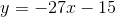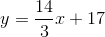Explanation:

Based on the information provided, you can find the slope of this line easily.  From that, you can use the point-slope form of the equation of a line to compute the line's full equation.  The slope is merely: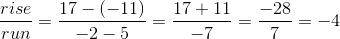Now, for a point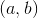and a slope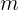, the point-slope form of a line is: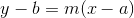Let's usefor our pointThis gives us: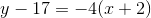Now, distribute and solve for: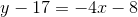### Example Question #59 : Coordinate Geometry

What is the equation of a line passing through the two points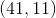and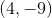?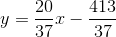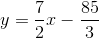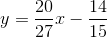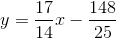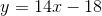Explanation:

Based on the information provided, you can find the slope of this line easily.  From that, you can use the point-slope form of the equation of a line to compute the line's full equation.  The slope is merely: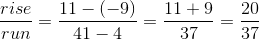Now, for a pointand a slope, the point-slope form of a line is:Let's usefor our pointThis gives us: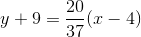Now, distribute and solve for: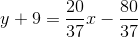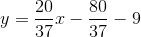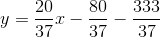### Example Question #60 : Coordinate Geometry

What is the equation of a line passing through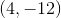with a-intercept of?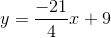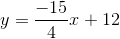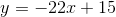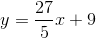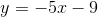Explanation:

Based on the information that you have been provided, you can quickly find the slope of your line.  Since the y-intercept is, you know that the line contains the point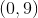.  Therefore, the slope of the line is found: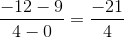Based on this information, you can use the standard slope-intercept form to find your equation:, where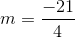and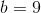### Example Question #1 : How To Find The Equation Of A Line

Given the graph of the line below, find the equation of the line.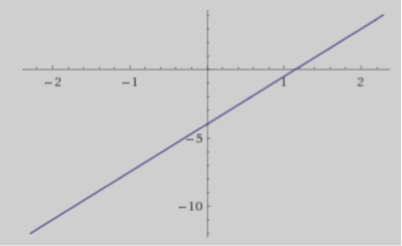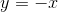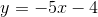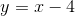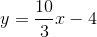Explanation:

To solve this question, you could use two points such as (1.2,0) and (0,-4) to calculate the slope which is 10/3 and then read the y-intercept off the graph, which is -4.

### Example Question #2 : How To Find The Equation Of A Line

Which line passes through the points (0, 6) and (4, 0)?

y = –3/2x + 6

y = 1/5x + 3

y = 2/3 + 5

y = –3/2 – 3

y = 2/3x –6

y = –3/2x + 6

Explanation:

P1 (0, 6) and P2 (4, 0)

First, calculate the slope:  m = rise ÷ run = (y2 – y1)/(x– x1), so m = –3/2

Second, plug the slope and one point into the slope-intercept formula:

y = mx + b, so 0 = –3/2(4) + b and b = 6

Thus, y = –3/2x + 6

### Example Question #3 : How To Find The Equation Of A Line

What line goes through the points (1, 3) and (3, 6)?

–2x + 2y = 3

2x – 3y = 5

–3x + 2y = 3

3x + 5y = 2

4x – 5y = 4

–3x + 2y = 3

Explanation:

If P1(1, 3) and P2(3, 6), then calculate the slope by m = rise/run = (y2 – y1)/(x2 – x1) = 3/2

Use the slope and one point to calculate the intercept using y = mx + b

Then convert the slope-intercept form into standard form.

### Example Question #4 : How To Find The Equation Of A Line

What is the slope-intercept form of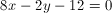?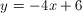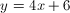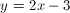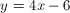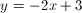Explanation:

The slope intercept form states that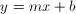. In order to convert the equation to the slope intercept form, isolate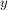on the left side: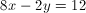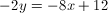### Example Question #5 : How To Find The Equation Of A Line

A line is defined by the following equation: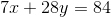What is the slope of that line?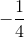Explanation:

The equation of a line is

y=mx + b where m is the slope

Rearrange the equation to match this:

7x + 28y = 84

28y = -7x + 84

y = -(7/28)x + 84/28

y = -(1/4)x + 3

m = -1/4

← Previous 1

Tired of practice problems?

Try live online GRE prep today.